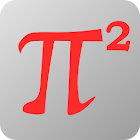Math2 - mathematics integrals

All Android applications categories

All Android games categories# Math2 - mathematics integrals

by: 376 7.8

7.8 Users
rating

## Screenshots

Description

Integrals on the phone!

Now you have all useful integral formulas handy and can always refer to them.

Range of material - University. Integrals for pros!

The content is divided into sections and subsections so that it is easy to find any formula or equation.

indefinite integrals
- Definitions
- Properties
- Basic formulas
- Integrals of rational functions
- Integrals of irrational functions
- Integrals of logarithmic functions
- Integrals of exponential functions
- Integrals of the sin functions

In preparation:
- Integrals of trigonometric functions
- definite integrals
- derivatives